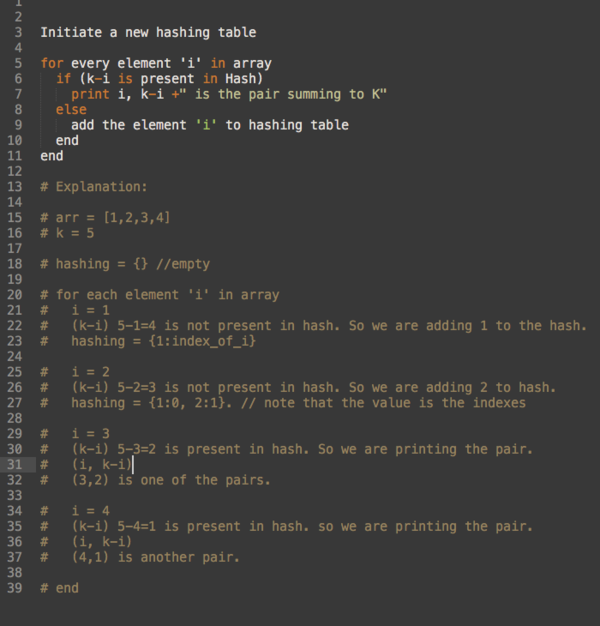Revue

# DS Weekly

November 14 · Issue #3 · View online
Learning data-structures and algorithms need commitment. I'm gonna make it easier by sending you a solved programming challenge weekly from a curated collection of data-structures, algorithms, competitive programming questions, interview questions straight to your mailbox.

Problem Statement
Consider an array of size n. And you are given a number k. Print all the pairs from the given array that sums to K.
Sample Input:
arr[1,2,3,4], k=5
Output:
(1,4)
(2,3)
Solution:
This problem is so simple. Before going into the problem let’s try to have a recap of what is Hash.
Hash is a data-structure used to store Key-Value pair. Most of you would know it but If you don’t know Hashing no problem you can take a look at this video
The solution is so simple. We gonna iterate through the array and for each element (i) in the array we gonna check the difference between k and the element (i) present in the hashing table or not.
If the difference (k-i) is present then it means that current element (i) and the difference (k-i) sums to form k. So we are printing the pair.
If the key is not present we are inserting the element (i) to the hashing table.
Hope you got it. If you didn’t, hold on.
PseudocodePseudocode
JavaJava
Here’s the link to the source code

If you got any feedback or anything you can reply to this email.
Thank you for reading this issue. :) :)
If you think this is helpful don’t mind to share it :) :)
Did you enjoy this issue?